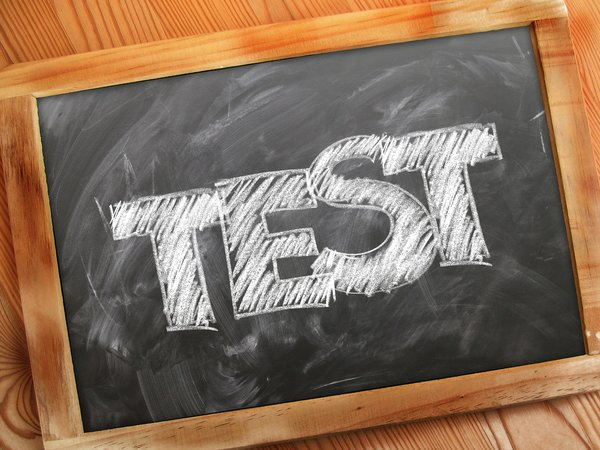# JEE Main 2020 Sample Question Paper With Solution For Numerical Value

The National Testing Agency (NTA) was vested with the authority to conduct the JEE Main exam. The Joint Entrance Examination (JEE) Main exam will be held twice a year in January and April. NTA has released the sample questions for the Numerical Value section, which will be conducted for the first time. The Agency has released the sample questions in numerical value - physics and chemistry. Aspirants who are aiming for the JEE Main in 2020 can access the sample question paper with the solution on the official website - jeemain.nta.nic.in.

JEE Main Question Papers

## JEE Main 2020 Sample Questions For Numerical Value (Physics)

Question 1: A particle moves in the x-y plane under the influence of a force such that the linear momentum Is P(t) = A [ î cos kt - ĵ sin kt ] Where A and k are constants. The angle in degrees between force and momentum is_________.

Question 2: A power line lies along the east-west direction and carries a current of 10 A. The force per meter due to earth's magnetic field of 10-4 T is 10-X N/m. The value of x is_____________.

Question 3: The sun's disc subtends an angle of 10-2 rad at the earth. The radius of curvature (in meters) of the mirror which will produce on a screen an image of the sun 2 cm in diameter is____________.

Question 4: The binding energy of deutron (1H2) is 1.15 MeV per nucleon and an alpha particle (2He4) has a binding energy of 7.1 MeV per nucleon. Then in the reaction 1H2+1H2→ 2He4+ Q The energy Q released in MeV is __________

Question 5: Light of wavelength 0.6 mm from a sodium lamp falls on a photo cell and causes the emission of photoelectrons for which the stopping potential is 0.5 V. With light of wavelength 0.4 mm from a mercury lamp the stopping potential is 1.5 V. Then, the work function in eV of the photocell surface is____________.

Question 1 - 90

Question 2 - 3

Question 3 - 4

Question 4 - 23.8

Question 5 - 1.5How To Start JEE Main Preparation

## JEE Main 2020 Sample Questions For Numerical Value (Chemistry)

Question 1: The hardness of a water sample (in terms of equivalents of CaCO3) containing 10-3M CaSO4 is : (molar mass of CaSO4 = 136g/mol).

Question 2: 50mL of 0.5M Oxalic Acid is needed to neutralize 25mL of sodium hydroxide solution. The amount of NaOH in 50mL of the given sodium hydroxide solution is ______________.

Question 3: The standard electrode potential Eº and its temperature coefficient (dEº/dT)p for a cell

are 2V and -5×10-4 VK-1 at 300K respectively. The cell reaction is Zn(s) +Cu2+(aq)→ Zn2+(aq) + Cu(s). The standard reaction enthalpy (ΔrHº) at 300K is _________________ kJ/mol (F= 96485 C mol-1).

Question 4: In the following reaction sequence, the mass percentage of carbon in the major product P is______________.

Question 5: The number of monochlorinated product obtained on chlorination of 2 methylbutane

is_________.

Question 1 - 100ppm

Question 2 - 2g

Question 3 - -414.9

Question 4 - 87.18%

Question 5 - 4

JEE Main will be conducted for three papers - BE/BTech, BArch and BPlan. A total of 20 questions will be of Multiple-Choice Questions (MCQs) and 5 questions will be as a Numerical Value. The JEE Main (January) 2020 session will be conducted from January 6 to 11. The JEE Main (April) will be held from April 3 to 9, 2020.How Students Should Register For Free JEE Main Mock Tests

Subscribe Now

--Or--
Select a Field of Study
Select a Course
Select UPSC Exam
Select IBPS Exam
Select Entrance Exam